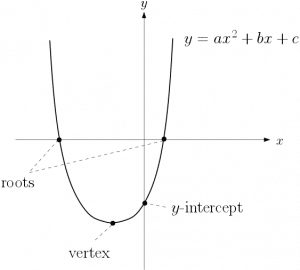# Pure Maths – fundamental principles and core toolsWe can think of Pure Maths as the fundamental principles and reasoning at the heart of any mathematics we do. It is the collection of underlying core tools required to explain and develop mathematical knowledge. This is as opposed to Applied Maths where we take knowledge of the pure mathematical subjects and apply it in real-life situations. For example, the study of functions, their features and their graphs, such as  quadratics, is Pure Mathematics. However, using quadratics to model the trajectory of a projectile is Applied Mathematics.

Pure Maths is thousands of years old. It was the ancient Greeks who first began to study maths as an organised science. Pythagoras, Euclid and Archimedes are just a few of the earliest known mathematicians – see the Mathematics Timeline.

In the AS Maths and A-Level Maths qualifications, Pure Maths makes up two-thirds of the content and hence the examinations. Click here to see more information on AS Maths exams or here for more A-Level Maths exam information. Also, see below for the topics studied in Pure Maths in AS and A-Level Mathematics.